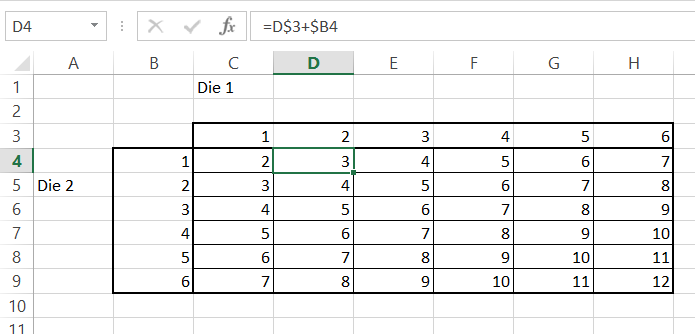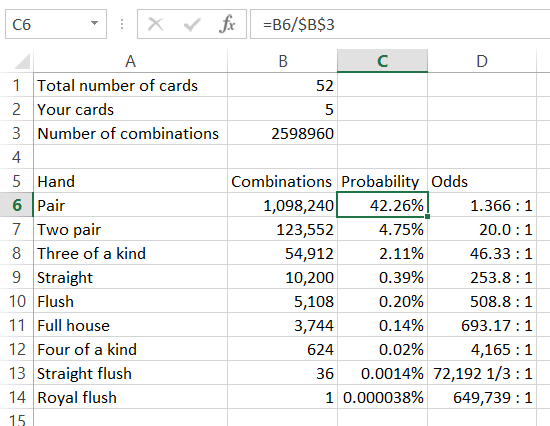## Calculating Probability in Excel

Probably you want to get to know how to calculate probability with Excel. You will after you take a look at this easy tutorial.

## Probability example

1. Enter data in Excel sheet.2. Use PROB function to calculate probability.Formula here is =PROB(A2:A10,B2:B10,B13,B14) where

• A2:A10 is range of marks in this example
• B2:B10 is a chance of getting the grade from A column
• B13 is lower range
• B14 stand for upper range

The probability here is 45%.

## 2 dice probability calculator

Let’s check what is the probability for rolling two dice.

I prepared data.First I need to calculate chance to roll.

It is possible to roll from 2 to 12 with two dice. The roll chance formula is: =COUNTIF(\$C\$4:\$H\$9,K4)

I used absolute reference in the formula.There are:

• only one chance to roll 2 and 12
• two chances to roll 3 and 11
• three chances to roll 4 and 10
• four chances to roll 5 and 9
• five chances to roll 6 and 8
• six chances to roll 7

To calculate 2 dice probability you need to divide the number of chances by sum of chances.

2 dice probability formula is =\$K4/SUM(\$K\$4:\$K\$14)Two dice probability is from 2.78% to 16.67%.

Based on above calculations you can easily calculate what is the probability of rolling doubles.

There are only 6 chances to do that so probability of rolling doubles is 6/36 = 16.67%. Interesting thing is that the probability of rolling doubles is exactly the same as to roll 7.

## Coin flip probability

It is 50% to flip head and 50% to flip tail. Have you ever wonder what is a probability to flip head (of tail) 11 times in a row? Let’s calculate it in Excel.

First let’s calculate probability to flip a head once. It is easy. 50%. Head or flip.

Translating it to Excel language we can create Excel formula: =POWER(0.5,1)

Going further probability is =POWER(0.5,number_of_tries)Probability to flip head (or tail) 11 times in a row equals 0.04883% which is less than 0.05%!

Flip a head 10 times is 0.09766% probable.

Flipping head (or tail) 20 times in a row is 0.000095% probable.

We can also check a chance to flip heads (or tails) times a row. It’s easy because each time is 2 times less probable.It’s 1 : 2048 odds to flip head (or tail) 11 times in a row. It means that every 2048 times you flip a coin 11 times you flip 11 heads (or tails).

## Poker probability

It will be fun to analyze poker probabilities.

First see the Excel function to check number of poker combinations. There are 52 cards from which you are getting only 5. From mathematic point of view it is simple combination to be used. There is an Excel function COMBIN for that. Function is taking just arguments of combination. Simply use Excel formula =COMBIN(52,5)Turns out there are 2,598,960 combinations in poker.

To calculate probability of getting pair or two pair you need to calculate the number of combinations of getting them. And then just divide two numbers.The probability is:

• To get a pair 42.26%
• To get two pair 4.75
• For a three of a kind 2.11%
• Straight 0.39%
• Full house 0.2%
• Four of a kind 0.14%
• Four of a kind 0.02%
• Straight flush 0.0014%
• Royal flush 0.000038% (1:649,736 odds)

## Template

```Further reading: